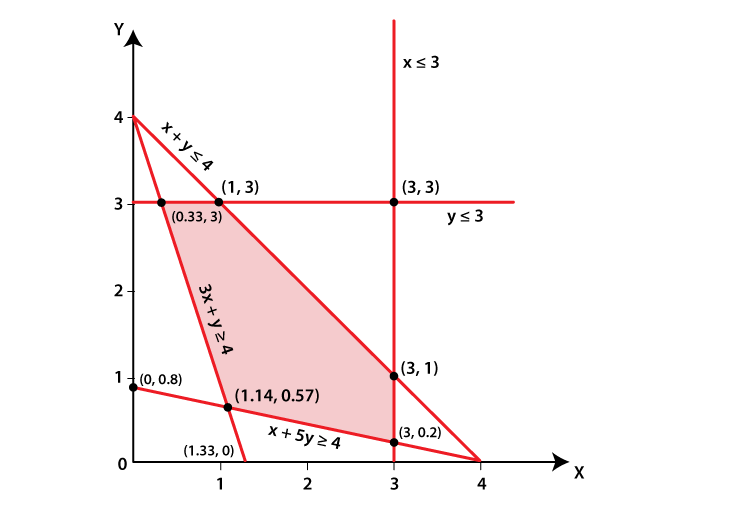# RD Sharma Solutions for Class 11 Chapter 15 - Linear Inequations Exercise 15.6

In this section, we will discuss the technique of finding the solution set of simultaneous linear inequations. Solving simultaneous linear inequations means finding the set of points (x,y) for which all the constraints are satisfied. The solutions pdf contains problems solved in various techniques to help students understand the steps to be followed in answering them. Important formulas and algorithms are discussed in simple language, in accordance with the understanding capacity of students. Here, students can find RD Sharma Class 11 Maths Solutions pdf, which can be downloaded effortlessly.

## Download the pdf of RD Sharma Solutions for Class 11 Maths Exercise 15.6 Chapter 15 – Linear Inequations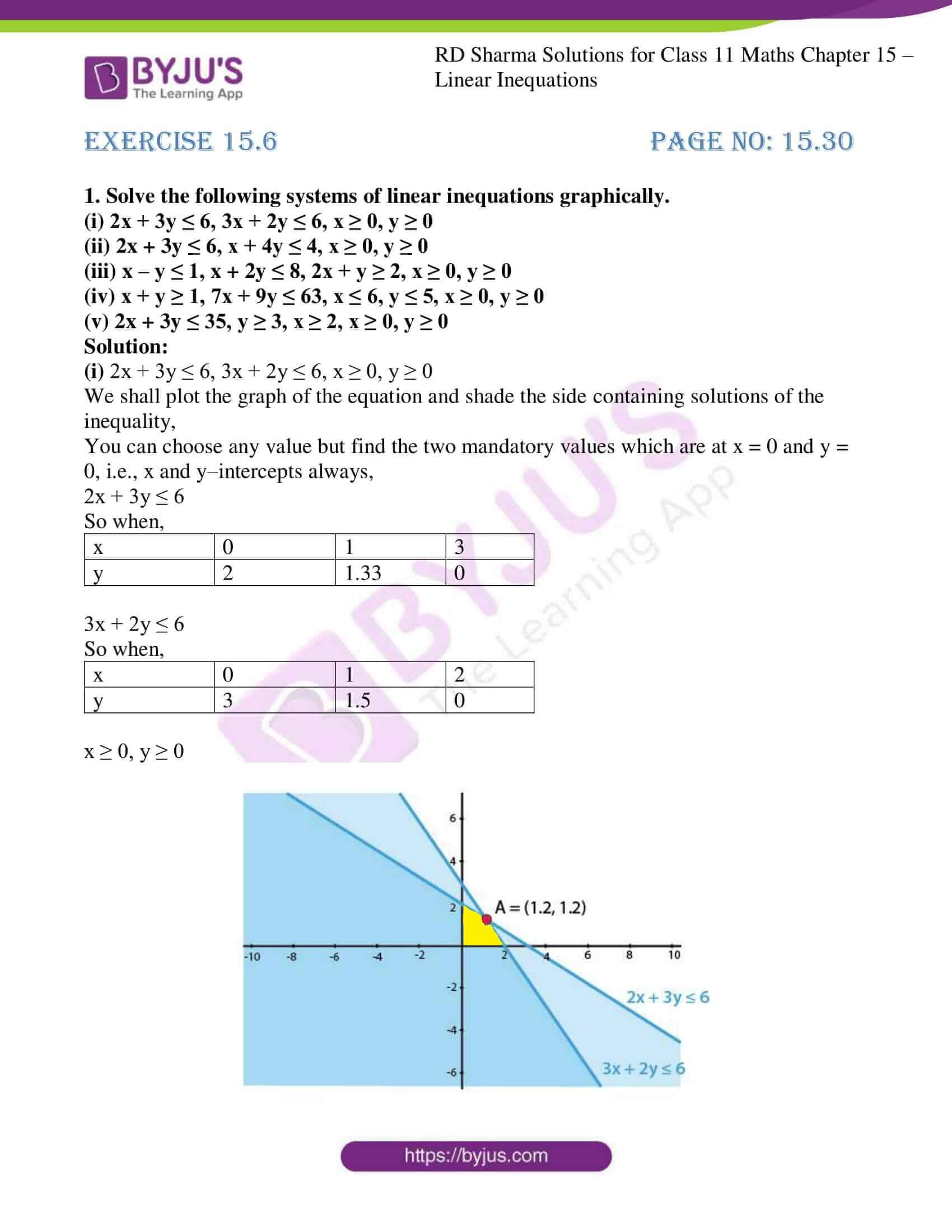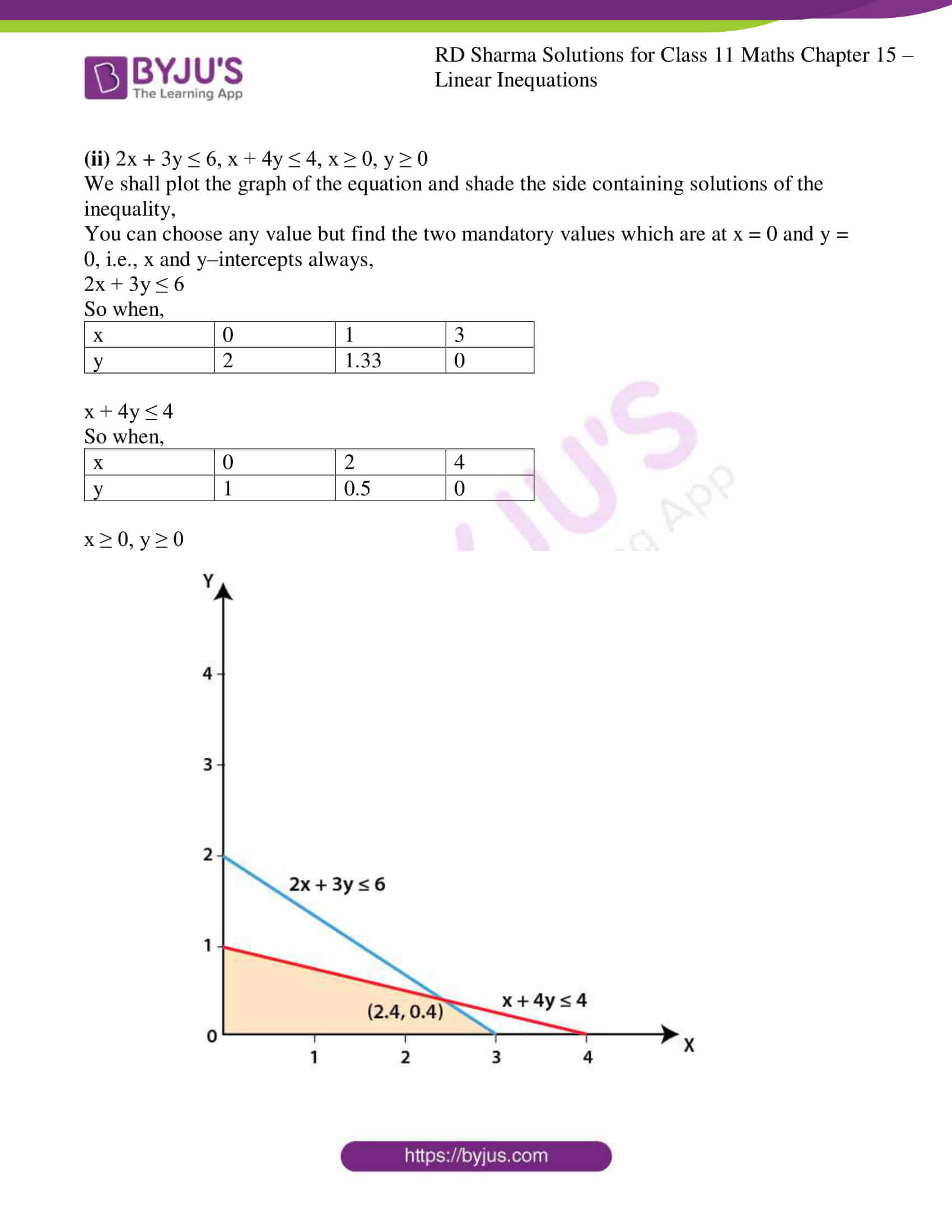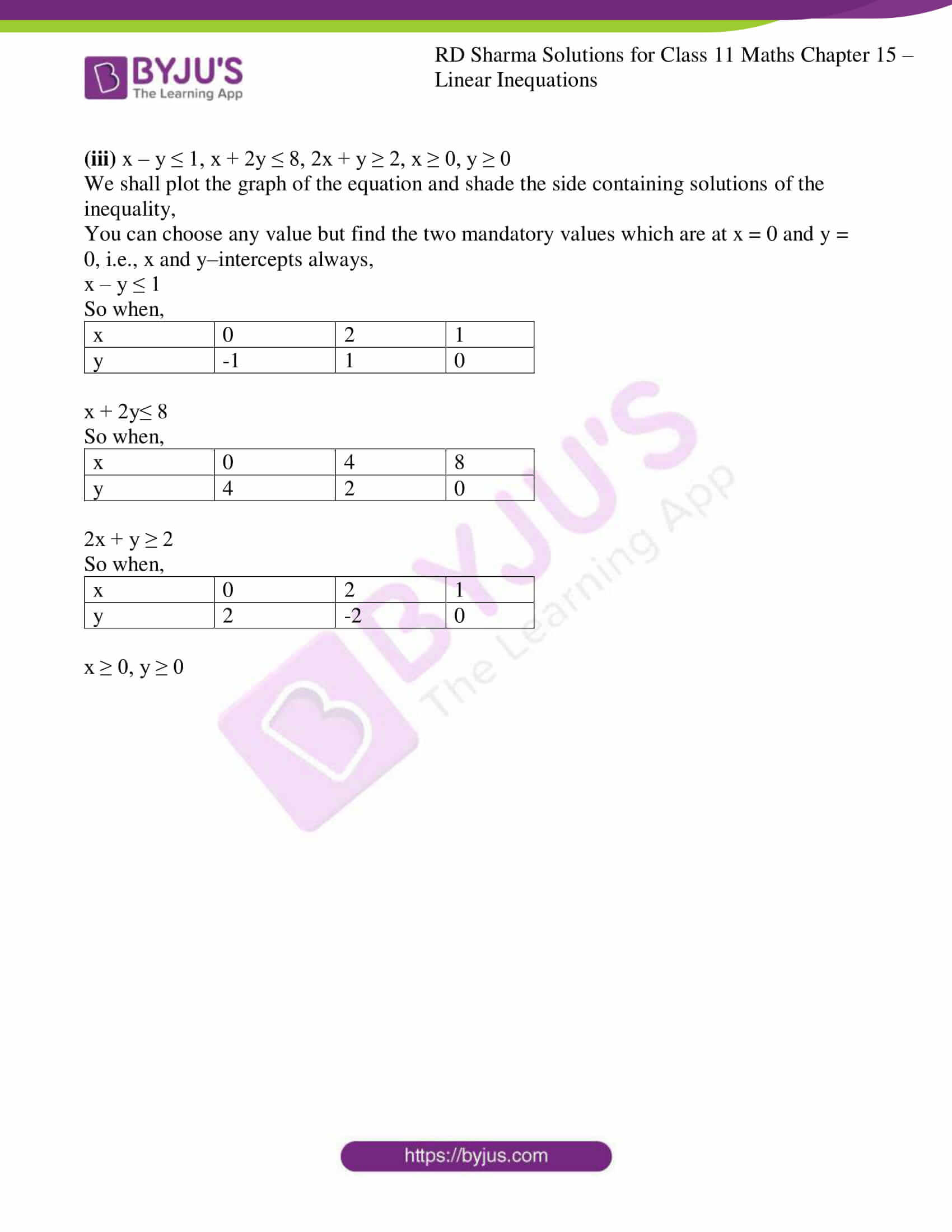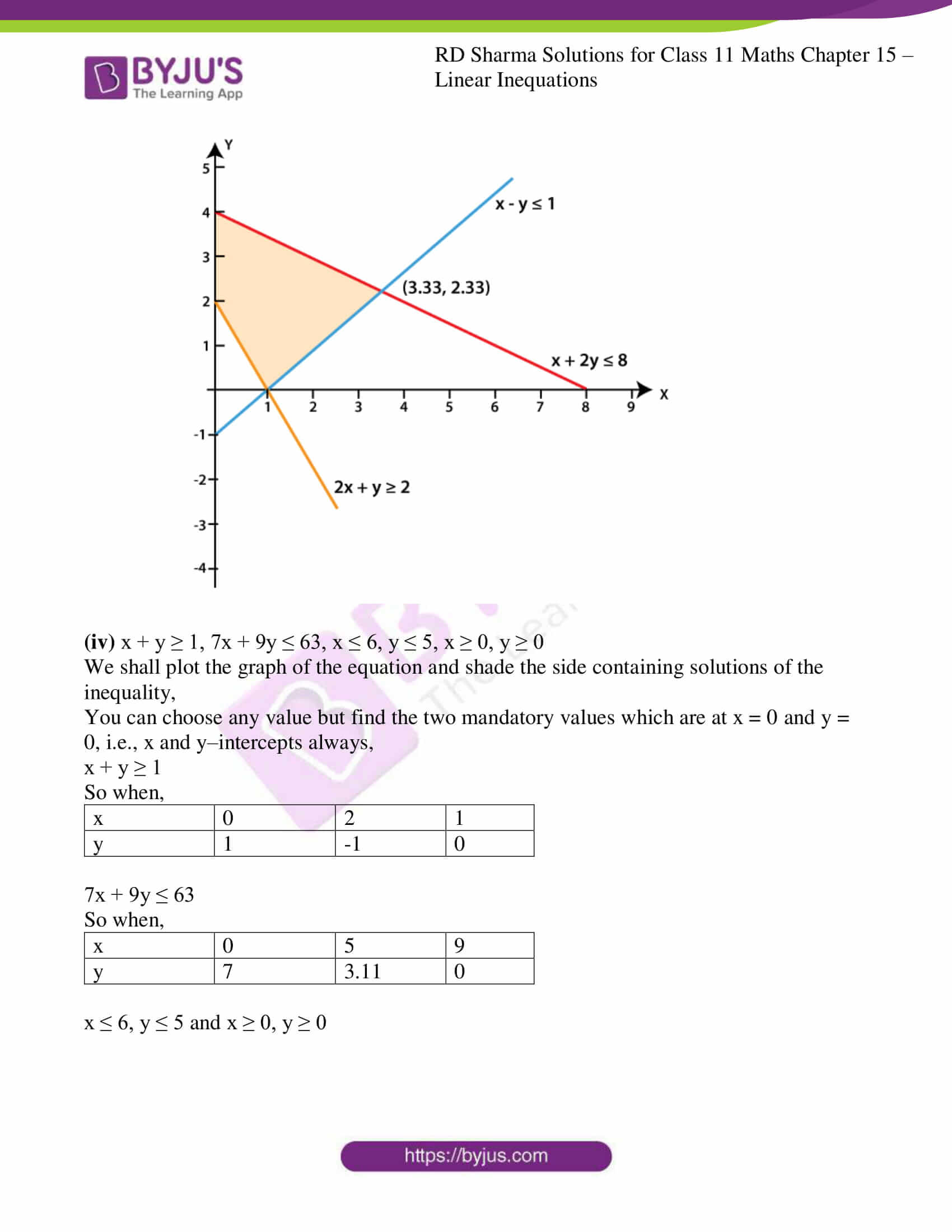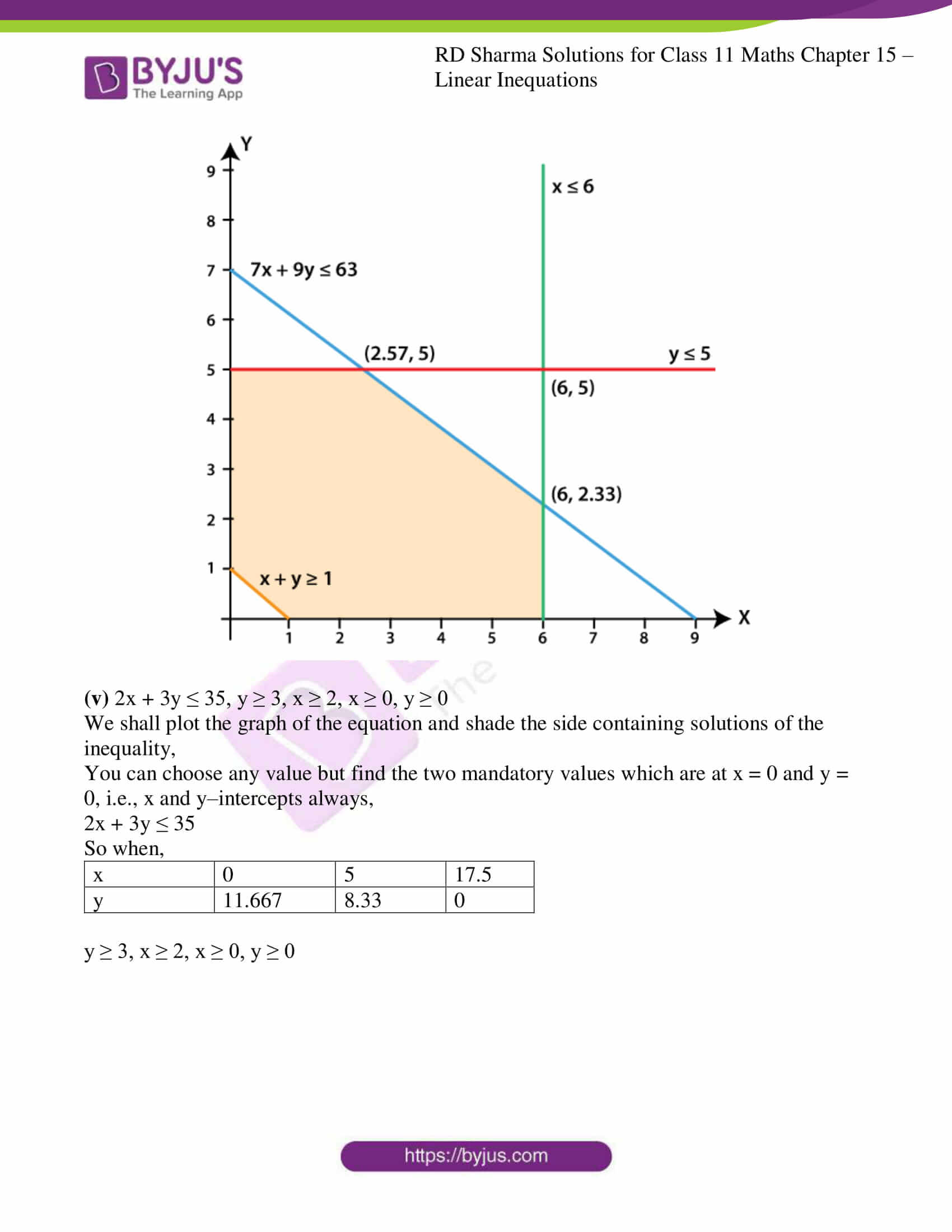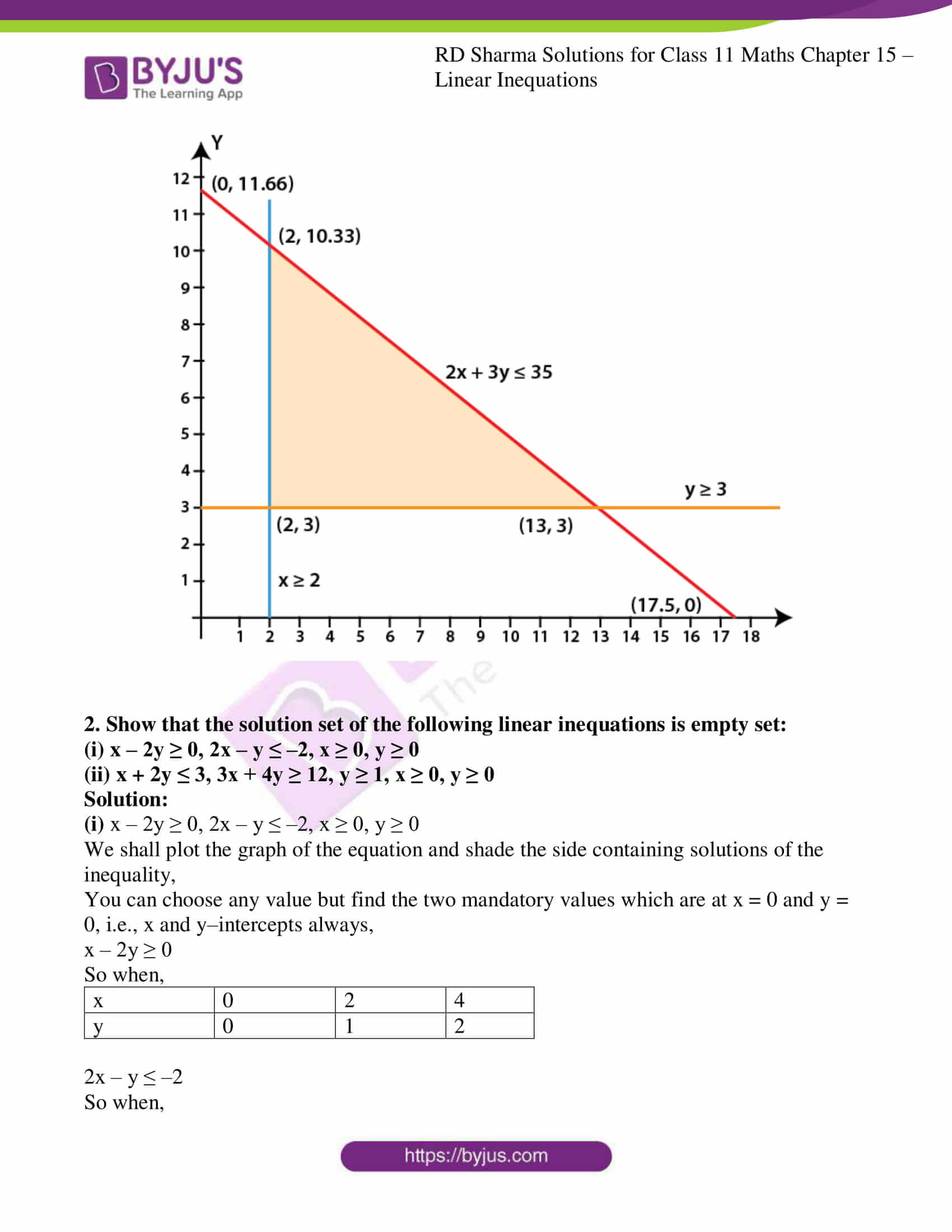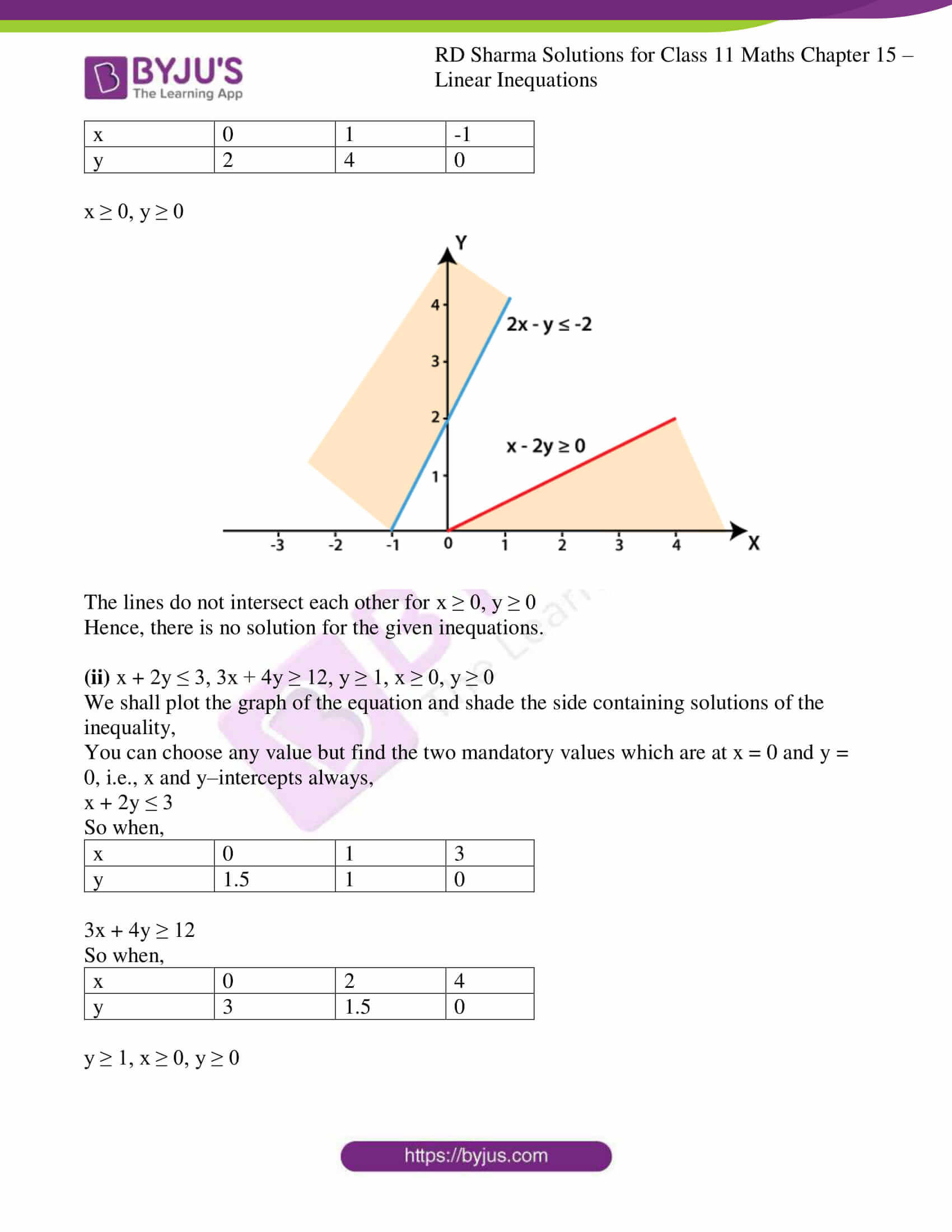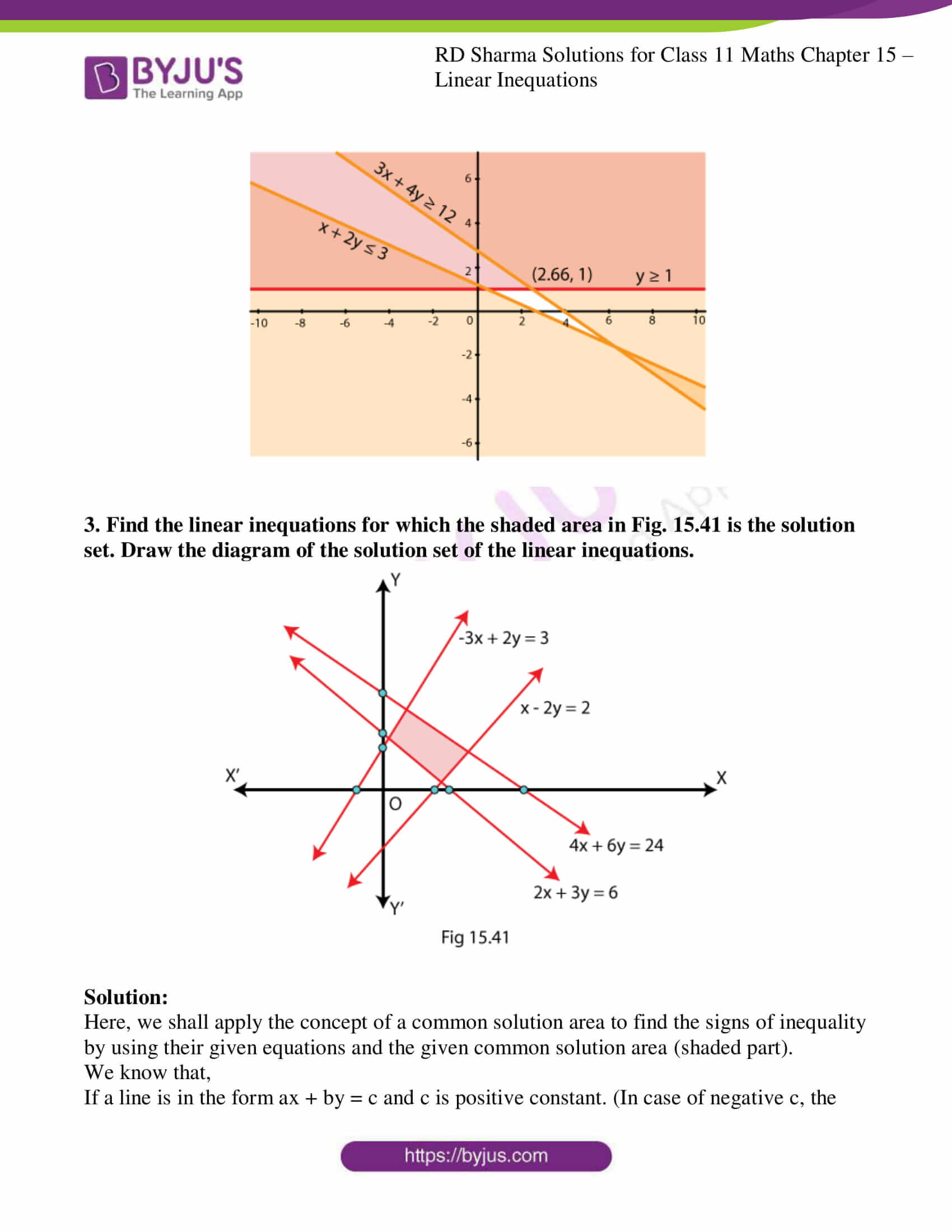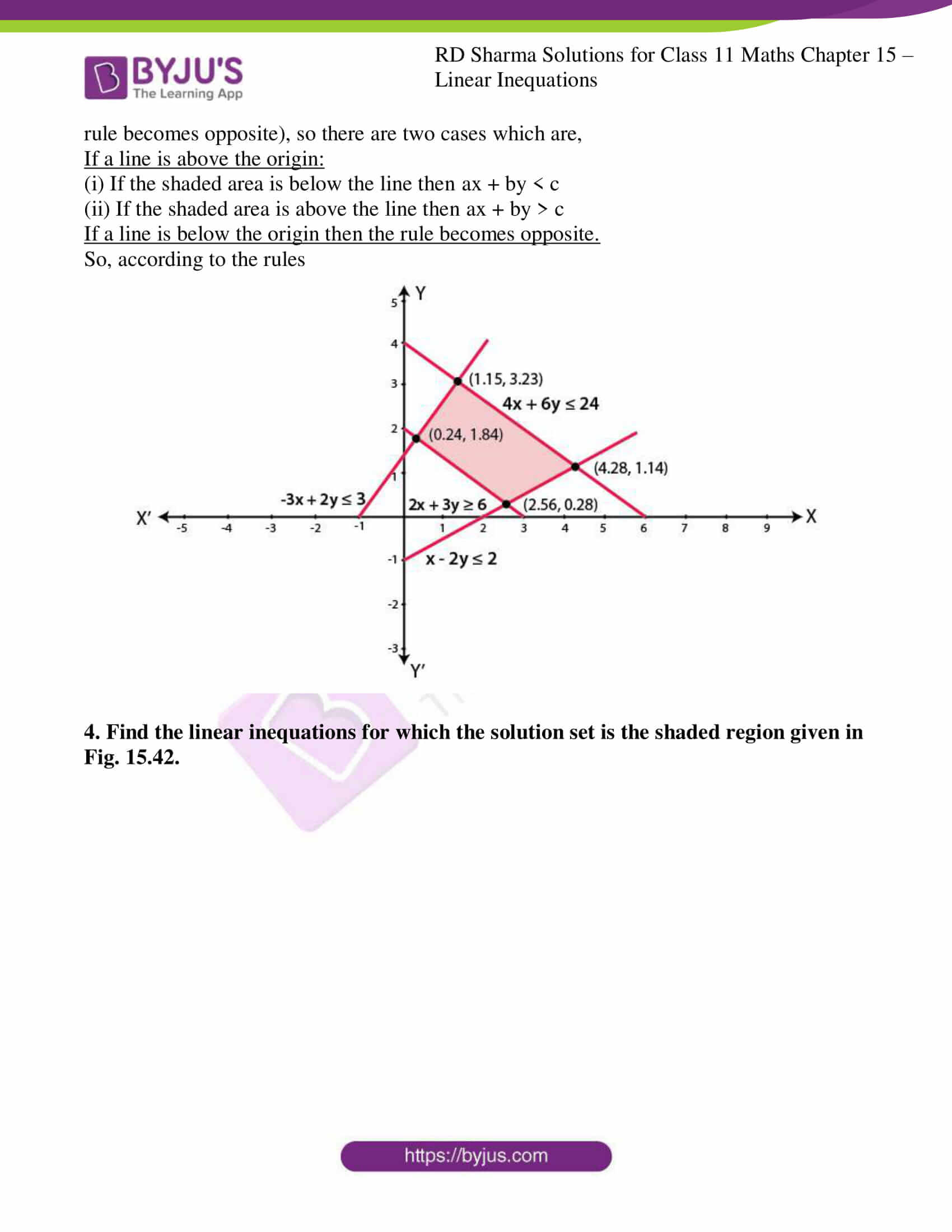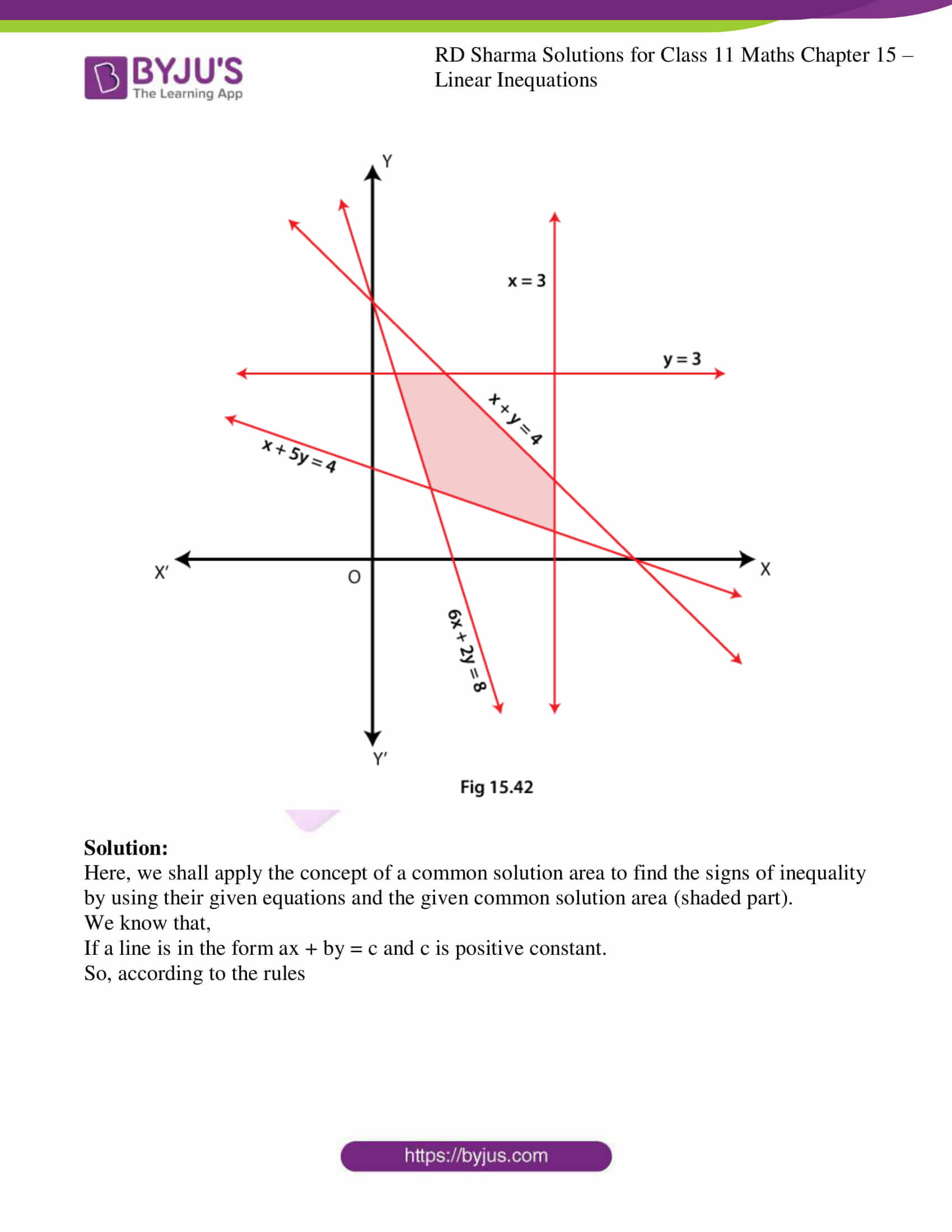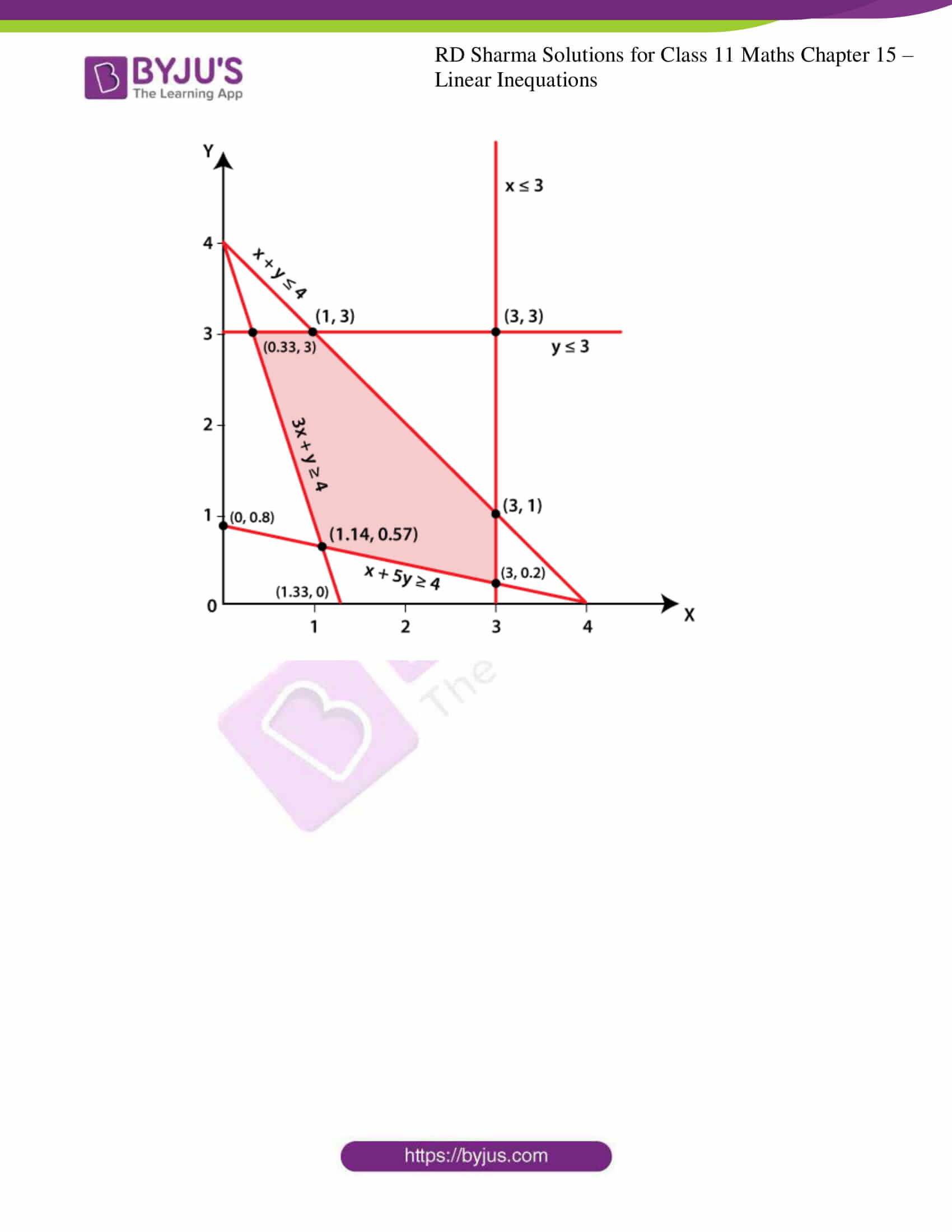### Also, access other exercises of RD Sharma Solutions for Class 11 Maths Chapter 15 – Linear Inequations

Exercise 15.1 Solutions

Exercise 15.2 Solutions

Exercise 15.3 Solutions

Exercise 15.4 Solutions

Exercise 15.5 Solutions

### Access answers to RD Sharma Solutions for Class 11 Maths Exercise 15.6 Chapter 15 – Linear Inequations

1. Solve the following systems of linear inequations graphically.
(i) 2x + 3y ≤ 6, 3x + 2y ≤ 6, x ≥ 0, y ≥ 0

(ii) 2x + 3y ≤ 6, x + 4y ≤ 4, x ≥ 0, y ≥ 0

(iii) x – y ≤ 1, x + 2y ≤ 8, 2x + y ≥ 2, x ≥ 0, y ≥ 0

(iv) x + y ≥ 1, 7x + 9y ≤ 63, x ≤ 6, y ≤ 5, x ≥ 0, y ≥ 0

(v) 2x + 3y ≤ 35, y ≥ 3, x ≥ 2, x ≥ 0, y ≥ 0

Solution:

(i) 2x + 3y ≤ 6, 3x + 2y ≤ 6, x ≥ 0, y ≥ 0

We shall plot the graph of the equation and shade the side containing solutions of the inequality,

You can choose any value but find the two mandatory values which are at x = 0 and y = 0, i.e., x and y–intercepts always,

2x + 3y ≤ 6

So when,

 x 0 1 3 y 2 1.33 0

3x + 2y ≤ 6

So when,

 x 0 1 2 y 3 1.5 0

x ≥ 0, y ≥ 0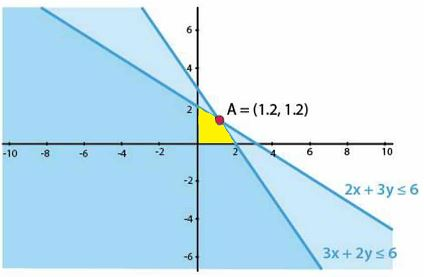(ii) 2x + 3y ≤ 6, x + 4y ≤ 4, x ≥ 0, y ≥ 0

We shall plot the graph of the equation and shade the side containing solutions of the inequality,

You can choose any value but find the two mandatory values which are at x = 0 and y = 0, i.e., x and y–intercepts always,

2x + 3y ≤ 6

So when,

 x 0 1 3 y 2 1.33 0

x + 4y ≤ 4

So when,

 x 0 2 4 y 1 0.5 0

x ≥ 0, y ≥ 0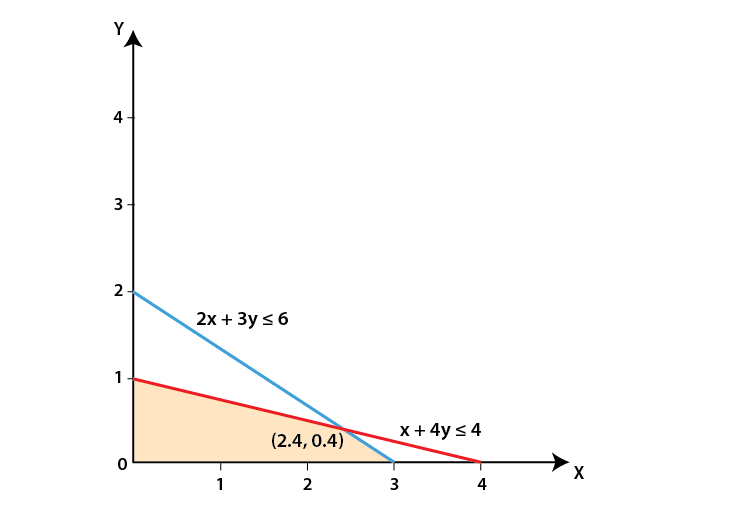(iii) x – y ≤ 1, x + 2y ≤ 8, 2x + y ≥ 2, x ≥ 0, y ≥ 0

We shall plot the graph of the equation and shade the side containing solutions of the inequality,

You can choose any value but find the two mandatory values which are at x = 0 and y = 0, i.e., x and y–intercepts always,

x – y ≤ 1

So when,

 x 0 2 1 y -1 1 0

x + 2y≤ 8

So when,

 x 0 4 8 y 4 2 0

2x + y ≥ 2

So when,

 x 0 2 1 y 2 -2 0

x ≥ 0, y ≥ 0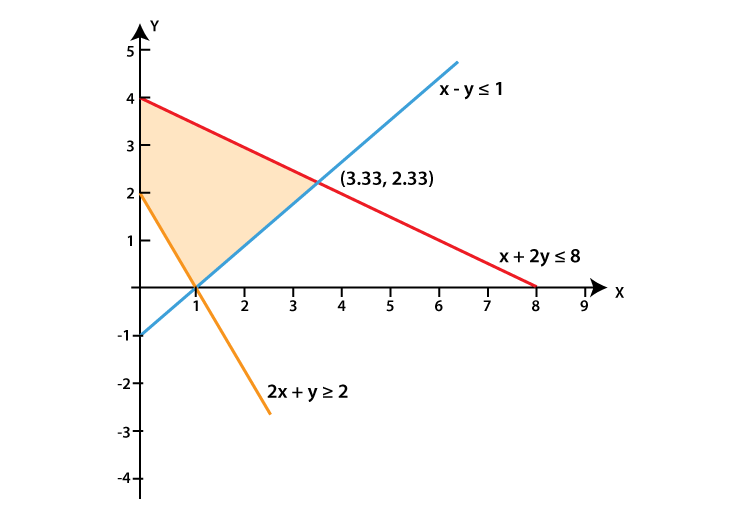(iv) x + y ≥ 1, 7x + 9y ≤ 63, x ≤ 6, y ≤ 5, x ≥ 0, y ≥ 0

We shall plot the graph of the equation and shade the side containing solutions of the inequality,

You can choose any value but find the two mandatory values which are at x = 0 and y = 0, i.e., x and y–intercepts always,

x + y ≥ 1

So when,

 x 0 2 1 y 1 -1 0

7x + 9y ≤ 63

So when,

 x 0 5 9 y 7 3.11 0

x ≤ 6, y ≤ 5 and x ≥ 0, y ≥ 0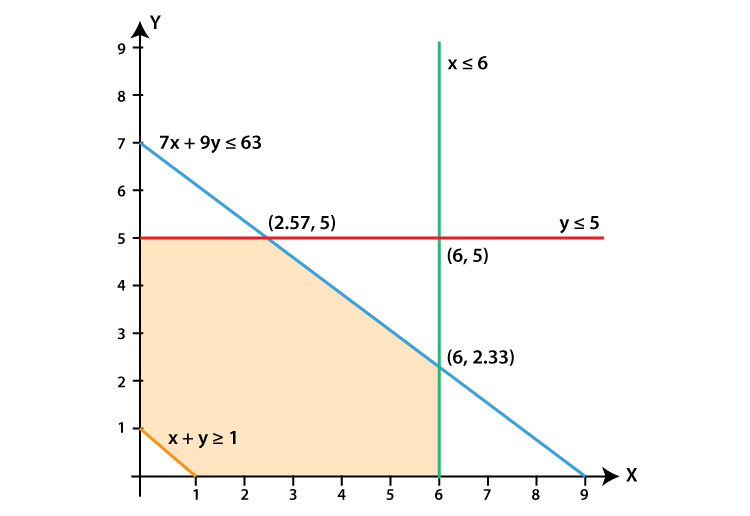(v) 2x + 3y ≤ 35, y ≥ 3, x ≥ 2, x ≥ 0, y ≥ 0

We shall plot the graph of the equation and shade the side containing solutions of the inequality,

You can choose any value but find the two mandatory values which are at x = 0 and y = 0, i.e., x and y–intercepts always,

2x + 3y ≤ 35

So when,

 x 0 5 17.5 y 11.667 8.33 0

y ≥ 3, x ≥ 2, x ≥ 0, y ≥ 0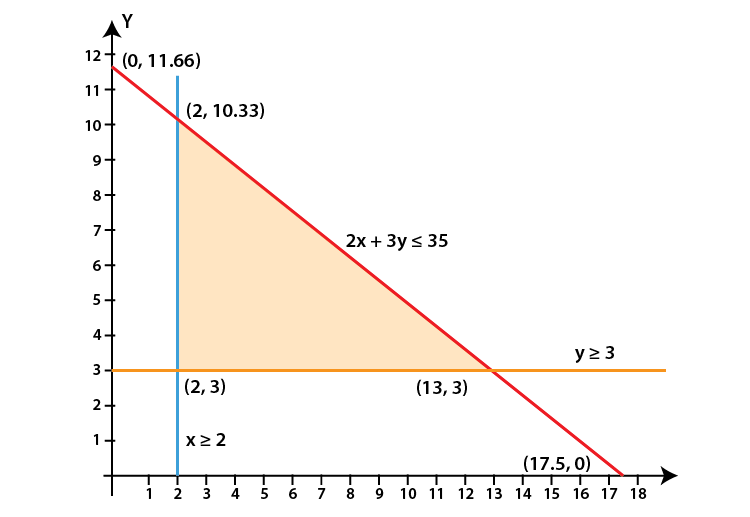2. Show that the solution set of the following linear inequations is empty set:
(i) x – 2y ≥ 0, 2x – y ≤ –2, x ≥ 0, y ≥ 0

(ii) x + 2y ≤ 3, 3x + 4y ≥ 12, y ≥ 1, x ≥ 0, y ≥ 0

Solution:

(i) x – 2y ≥ 0, 2x – y ≤ –2, x ≥ 0, y ≥ 0

We shall plot the graph of the equation and shade the side containing solutions of the inequality,

You can choose any value but find the two mandatory values which are at x = 0 and y = 0, i.e., x and y–intercepts always,

x – 2y ≥ 0

So when,

 x 0 2 4 y 0 1 2

2x – y ≤ –2

So when,

 x 0 1 -1 y 2 4 0

x ≥ 0, y ≥ 0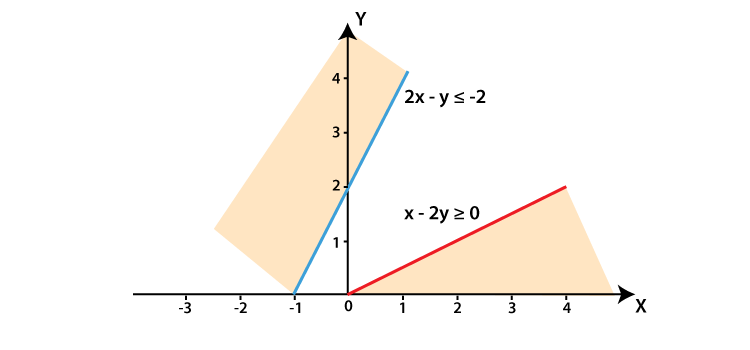The lines do not intersect each other for x ≥ 0, y ≥ 0

Hence, there is no solution for the given inequations.

(ii) x + 2y ≤ 3, 3x + 4y ≥ 12, y ≥ 1, x ≥ 0, y ≥ 0

We shall plot the graph of the equation and shade the side containing solutions of the inequality,

You can choose any value but find the two mandatory values which are at x = 0 and y = 0, i.e., x and y–intercepts always,

x + 2y ≤ 3

So when,

 x 0 1 3 y 1.5 1 0

3x + 4y ≥ 12

So when,

 x 0 2 4 y 3 1.5 0

y ≥ 1, x ≥ 0, y ≥ 0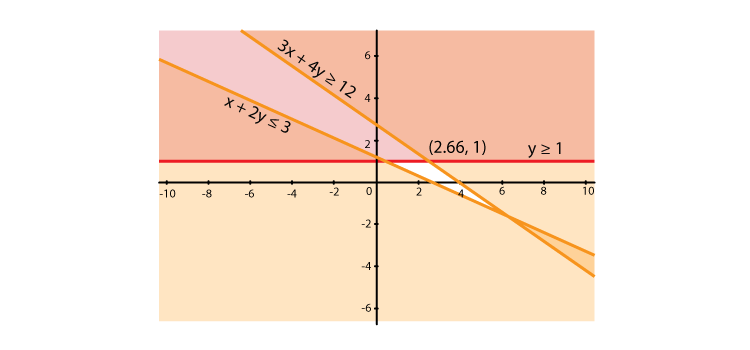3. Find the linear inequations for which the shaded area in Fig. 15.41 is the solution set. Draw the diagram of the solution set of the linear inequations.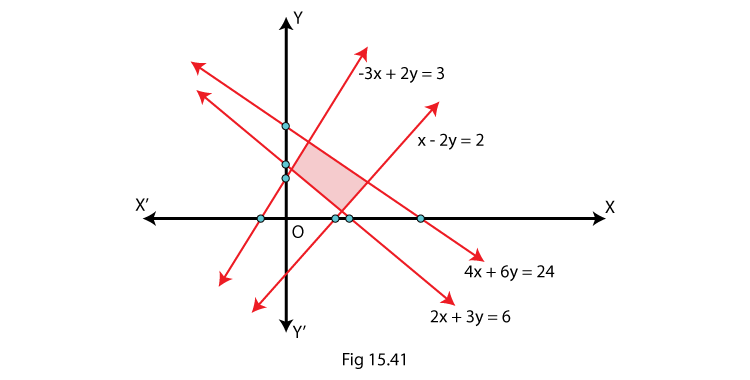Solution:

Here, we shall apply the concept of a common solution area to find the signs of inequality by using their given equations and the given common solution area (shaded part).

We know that,

If a line is in the form ax + by = c and c is positive constant. (In case of negative c, the rule becomes opposite), so there are two cases which are,

If a line is above the origin:

(i) If the shaded area is below the line then ax + by < c

(ii) If the shaded area is above the line then ax + by > c

If a line is below the origin then the rule becomes opposite.

So, according to the rules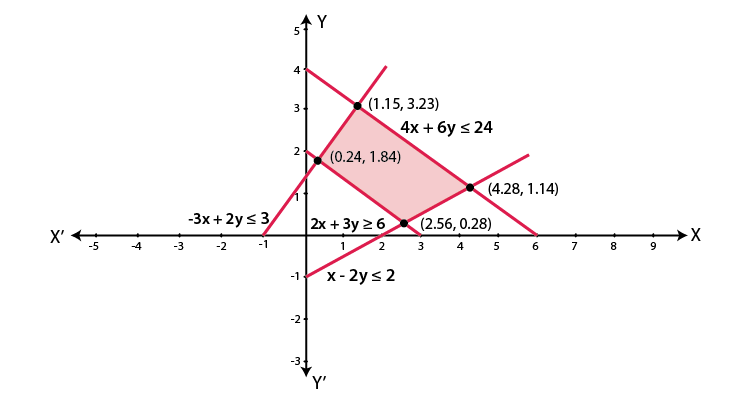4. Find the linear inequations for which the solution set is the shaded region given in Fig. 15.42.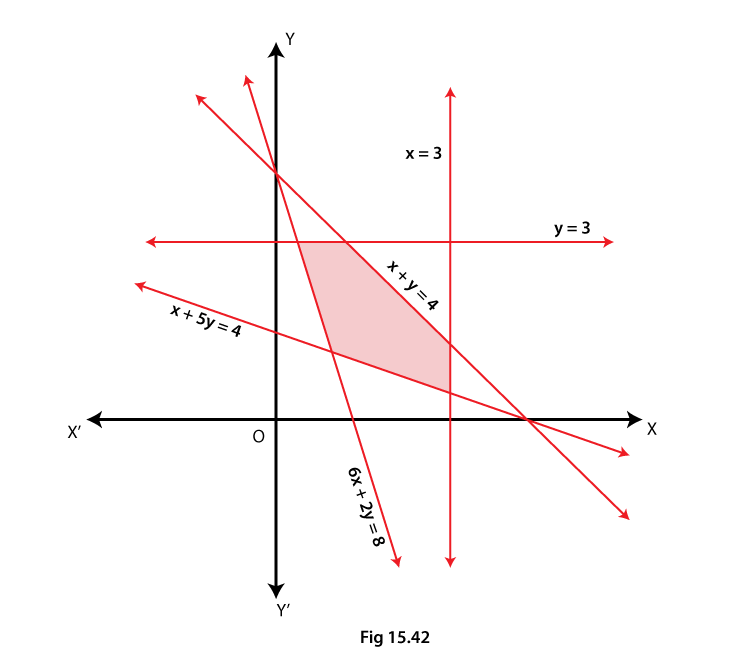Solution:

Here, we shall apply the concept of a common solution area to find the signs of inequality by using their given equations and the given common solution area (shaded part).

We know that,

If a line is in the form ax + by = c and c is positive constant.

So, according to the rules